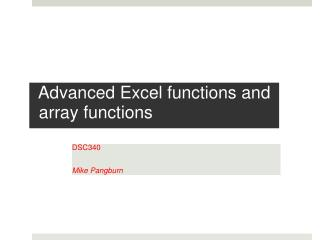DownloadDownload PresentationAdvanced Excel functions and array functions

# Advanced Excel functions and array functions

Download Presentation## Advanced Excel functions and array functions

- - - - - - - - - - - - - - - - - - - - - - - - - - - E N D - - - - - - - - - - - - - - - - - - - - - - - - - - -
##### Presentation Transcript

1. Advanced Excel functions andarray functions DSC340 Mike Pangburn

2. POA • Useful standard functions • Text and logic functions • “Lookup” functions • Conditional counting / summing • Array functions

3. Nice tip when using any formulae in Excel • You can toggle between the “results view” and “formula view” in Excel by clicking the key combinationCTRL- ` (The ctrl key and the left single quote, together)

4. Excel text functions • Functions that return part of the text within a text cell • LEFT(text, num_chars) • RIGHT(text, num_chars) • MID(text, start_num, num_chars) • Function that identifies the starting position of some text within a cell • FIND(find_text, within_text)

5. Excel text functions in action • Goal: extract the username and domain strings from the email address (in cell A2). • You may have a list of 1000’s of email addresses to go through… need to use functions to get it done

6. Excel text functions • Concatenating cells’ text • Two options • =CONCATENATE • &

7. Excel logic functions • The core logic functions are • AND • =AND(“T/F condition”, “T/F condition”) • OR • =OR(“T/F condition”, “T/F condition”) • NOT • =NOT(“T/F condition”) • IF • =IF(“T/F condition”, true-result, false-result) • Let’s look at some examples in the accompanying spreadsheet

8. Excel “Lookup” functions • Useful functions for finding values in a range of cells include: • LOOKUP() • VLOOKUP() • HLOOKUP() • You should know these from prior work with Excel

9. A different approach for finding a particular cell: the OFFSET function • OFFSET • Allows you to return the value of any cell by specifying its location in your spreadsheet relative to another cell. • Syntax: • =offset(reference_cell, rows_down, cols_right)

10. OFFSET function example

11. Conditional counting/summing • Example: Count how many “Jan” sales months there are. • =COUNTIF(A2:A10,"Jan”) • Example: compute total sales across all “Jan” months. • =SUMIF(A2:A10,"Jan",C2:C10) • Nice trick: Compute total sales where month is “Jan” or “Mar.” • =SUMIF(A2:A10,"Jan",C2:C10) + SUMIF(A2:A10,“Mar”,C2:C10)

12. POA • Useful standard functions • Text and logic functions • “Lookup” functions • Conditional counting / summing • Array functions

13. Excel range calculations (often called “array functions”) • Normally, you think about calculations in terms of working with two “single cells” • But often in spreadsheet work, you have calculations that span entire ranges • Such are called arraytype calculations • To signal to Excel that your calculation is an array type calculation, it use CTRL-SHIFT-RETURN instead of just hitting the RETURN key • Array calculations can involve standard functions: • e.g., *, COUNTIF • …there are also specific Excel array functions: • =FREQUENCY()

14. Excel range calculations “array functions” • Example: Compute total sales where month is “Jan” andregion is “North” • Can we use our last COUNTIF “trick?” No, the conditions must be in the same field. • Array function: when you enter this formula, use Ctrl+Shift+Enter • =SUM((A2:A10="Jan") * (B2:B10="North") * C2:C10) Multiplying 0 and 1 values

15. Excel range calculations “array functions” • Example: Compute total sales where month is “Jan” andregion is not “North” • Array function: • =SUM((A2:A10="Jan") * (B2:B10<>"North") * C2:C10) • Example:Count the number of sales months where month is “Jan” and region is not “North” • =SUM((A2:A10="Jan") * (B2:B10<>"North”)) (array fn) Multiplying 0 and 1 values

16. Excel range calculations “array functions” • Example: Compute total sales where month is “Jan” andthe sales value is 200 or more. • Array function: • =SUM((A2:A10="Jan") * (C2:C10>=200) * C2:C10) • Example:Comput total sales that are between 300 and 400. • Array function: • =SUM((C2:C10>=300)*(C2:C10<=400)*(C2:C10))